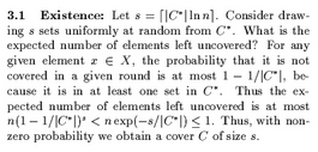## neal young / Young95Randomized

•Randomized rounding is a standard method, based on the probabilistic method, for designing combinatorial approximation algorithms. This paper introduces a variant of randomized rounding that can be used to derive Lagrangian-relaxation algorithms and greedy algorithms. This was surprising - the approximation algorithms derived previously by the method require first solving a linear program (or some other optimization problem). Indeed, in Prabhakar Raghavan's seminal paper introducing randomized rounding , he says: "The time taken to solve the linear program relaxations of the integer programs dominates the net running time theoretically (and, most likely, in practice as well)."

The variant of randomized rounding described here avoids this bottleneck, at least for packing/covering-type problems. The resulting algorithms are Lagrangian-relaxation algorithms and greedy algorithms (in fact the greedy set-cover algorithm is an example), and are faster and simpler to implement than standard randomized-rounding algorithms. This approach gives a systematic, coherent, and comprehensive understanding of Lagrangian-relaxation algorithms for packing and covering linear programs.

The ideas introduced here are also used in Sequential and parallel algorithms for mixed packing and covering'' , in K-medians, facility location, and the Chernoff-Wald bound'' ), and in On the number of iterations for Dantzig-Wolfe optimization and packing-covering approximation algorithms'' .
Lecture notes on this topic are here.